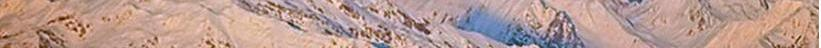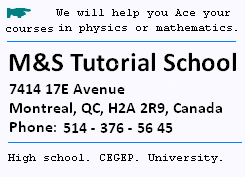Contents

© The scientific sentence. 2010# Total enegy of any particle

### 1. Preliminary

#### 1. The Binomial series

(1 + x)n can be written as the Taylor series, that is:
(1 + x)n = 1 + nx +(1/2)n(n - 1)x2 + ...
when |x| < 1.

#### 2. Total energy:

E = mc2 = γm0c2 =
m0c2/[1 - u2/c2]1/2 =
m0c2 [1 - u2/c2]- 1/2
Here, in our case, x = - u2/c2, n = - 1/2, and v << c ( the velocity of the particle is very
smaller that c is). Therefore:

E = m0c2 [1 + (1/2)u2/c2 + (3/8)u4/c4 + ... ]
= m0c2 + m0c2[(1/2)u2/c2 + (3/8)u4/c4 + ... ] = E0 + KE,
with:
E0 = m0c2, the energy at rest, and
KE = m0c2[ (1/2)u2/c2 + (3/8)u4/c4 + ... ]
the kinetic energy of the prticle.

The more we go on the terms of the series, the less these terms significant become. We disregard them from the second term for the linear momentum and from the third one regarding the total energy, and write at the first order:

KE = m0c2 (1/2)u2/c2 = (1/2) m0u2
KE = (1/2)m0u2
We find the well known results from Classical Mechanics.

E0 + KE means that to move a particle, it's necessary at first to have at least its energy at rest E0 = m0c2.
Even at rest, an object has energy, that is E0 = m0c2, Mass is energy.

#### 3. The relativistic linear momentum

The relativistic linear momentum is P = mu
m is the relativistic mass (= γm0) and u is the velocity of the particle) m and u are measured in the rest frame.
γ = 1/[1 - u2/c2]1/2.

### 2.Total energy and the Linear momentum

We are interested here to find the relation between the total energy E and the relativistic Linear momentum P.
γ = 1/[1 - u2/cγ = 1/[1 - u2/c2]1/2]1/2
1/ (γ2 - 1 ) = c22u2
E2 = m2c4 = P2c4/u2
= P2c2. c2/u2
c2/u2 = γ2/(γ2 - 1)
Then:
E2 = P2c2[(γ2 - 1 + 1)/γ2 - 1]
= P2c2[1 + 1/(γ2 - 1)]
P2c2/(γ2 - 1) = m02c4
It follows that:
E2 = P2c2 + m02c4

E2 = P2c2 + m02c4

#### The case of photons

When the velocity of a particle approches the value of c, γ becomes infinite and its related mass as well. The mass becomes infinite does not mean that it weights infinity!. It just mean that when a particle moves faster and its speed approches c, its inertial mass becomes strong enough to be stopped.

For a photon, the mass at rest in null, then: E = P c

For low speeds, v/c << 1, we have:
P = m0u (classic)
E2 = m02u2 + m02c4 = m02c4[1 + u2/c2].
Therefore:
E = m0c2[1 + u2/2c2] = m0c2 + (1/2)m0u2

For a photon:

E = P c = hν = hc/λ

 chimie labs | Physics and Measurements | Probability & Statistics | Combinatorics - Probability | Chimie | Optics | contact |